Background: SIMD and Auto-Vectorization

Modern CPU’s have a variety of SIMD (single input multiple data) vector instructions (eg. intel’s `SSE` and `AVX`, ARM’s `NEON` and `SVE`). They make use of vector registers, which can hold multiple values of a type. For example a `avx512` registers (512 bit) can hold 64 bytes, or 16 ints/floats, or 8 long/doubles. They can thus load, store, add, multiply, etc multiple values with a single instruction, but usually at the same cost (instructions per cycle, and latency) as with scalar (single) values.

It is thus beneficial to use SIMD instructions rather than their scalar equivalent. We can do this by hand.

``````    static void test(float[] data) {
for (int j = 0; j < N; j++) {
data[j] = 2 * data[j]; // ------------ scalar
}
}
static void test_unrolled(float[] data) {
// Pre loop
for (int j = 0; j < N; j+=4) { // increment by 4 elements at a time
data[j + 0] = 2 * data[j + 0];
data[j + 1] = 2 * data[j + 1];
data[j + 2] = 2 * data[j + 2];
data[j + 3] = 2 * data[j + 3];
}
// Post loop
}
static void test_vectorized(float[] data) {
// Pre loop
for (int j = 0; j < N; j+=4) { // increment by 4 elements at a time
vector_4floats v1 = data[j : j + 4]; // vector load
vector_4floats v2 = vector_4floats(2, 2, 2, 2); // vector constant
vector_4floats v3 = v1 * v2 // element-wise: 4 parallel multiplications
data[j : j + 4] = v3; // vector store
}
// Post loop
}
``````

I am not going into the details of Pre-Main-Post loops (Pre-loop ensures that the Main-loop is memory-aligned, the Post-loop executes the few iterations left over after the Main-loop).

While we can do this work by hand, we do not want to do that. For one, it is a lot of programming effort. Second, the vector length depends on the concrete CPU features, so one would have to have different vectorized code for each CPU. Hence, we want to have an algorithm in the compiler that does that work for us. It is supposed to detect where SIMD parallelization is possible, and decide if it is beneficial for performance. The primary concern is usually loops, where often most of the time is spent. But in principle one could speed up any part of a program that has enough parallelism.

The SuperWord Paper

In 2000, Samuel Larsen and Saman Amarasinghe presented Exploiting Superword Level Parallelism with Multimedia Instruction Sets. They auto-vectorize basic blocks, so that multiple scalar operations can be packed into a SIMD vector instruction. Their algorithm can thus be used on any program part that does not contain control flow (no branching / merging, no If, etc). The only requirement is that it has sufficient parallelism. For loops, this can often be achieved by loop unrolling. That way, one can fuse together multiple loop iterations into one basic block, and exploit the potential parallelism between different loop iterations.

The algorithm has been implemented in the HotSpot JVM. However, there are a few things that have been altered for the implementation. It only applies the algorithm to loop bodies, after a few iterations of loop unrolling.

First Example

Let’s look at a simple Java example (`Test.java`):

``````public class Test {
static int N = 100;

public static void main(String[] strArr) {
float[] data0 = new float[N];
for (int i = 0; i < 10_000; i++){
init(data0);
test(data0);
}
}

static void test(float[] data) {
for (int j = 0; j < N; j++) {
data[j] = 2 * data[j]; // <-- this is vectorized
}
}

static void init(float[] data) {
for (int j = 0; j < N; j++) {
data[j] = j;
}
}
}
``````

Execute it like this (with a debug build), and you should see that `SuperWord` was performed, among many other loop optimizations:

``````./java -XX:CompileCommand=printcompilation,Test::test -XX:CompileCommand=compileonly,Test::test -Xbatch -XX:+TraceLoopOpts Test.java
``````

Run this to see more details about the SuperWord algorithm, and its steps:

``````./java -XX:CompileCommand=printcompilation,Test::test -XX:CompileCommand=compileonly,Test::test -Xbatch -XX:+TraceSuperWord Test.java
``````

I ran it with this command, and recorded it with `rr`:

``````// -XX:LoopMaxUnroll=5 limits the unrolling factor to 4x.
rr ./java -XX:CompileCommand=printcompilation,Test::test -XX:CompileCommand=compileonly,Test::test -Xbatch -XX:+TraceSuperWord -XX:+TraceLoopOpts -XX:LoopMaxUnroll=5 Test.java
rr replay

// Set breakpoint before SuperWord is applied:
(rr) b SuperWord::SLP_extract

// Extract all nodes of the loop before SuperWord is applied:

// Run SuperWord:
(rr) finish

// Extract all nodes of loop after SuperWord:
(rr) p cl->dump_bfs(1000,cl, "#A+\$")
``````

The two dumps I visualize nicely: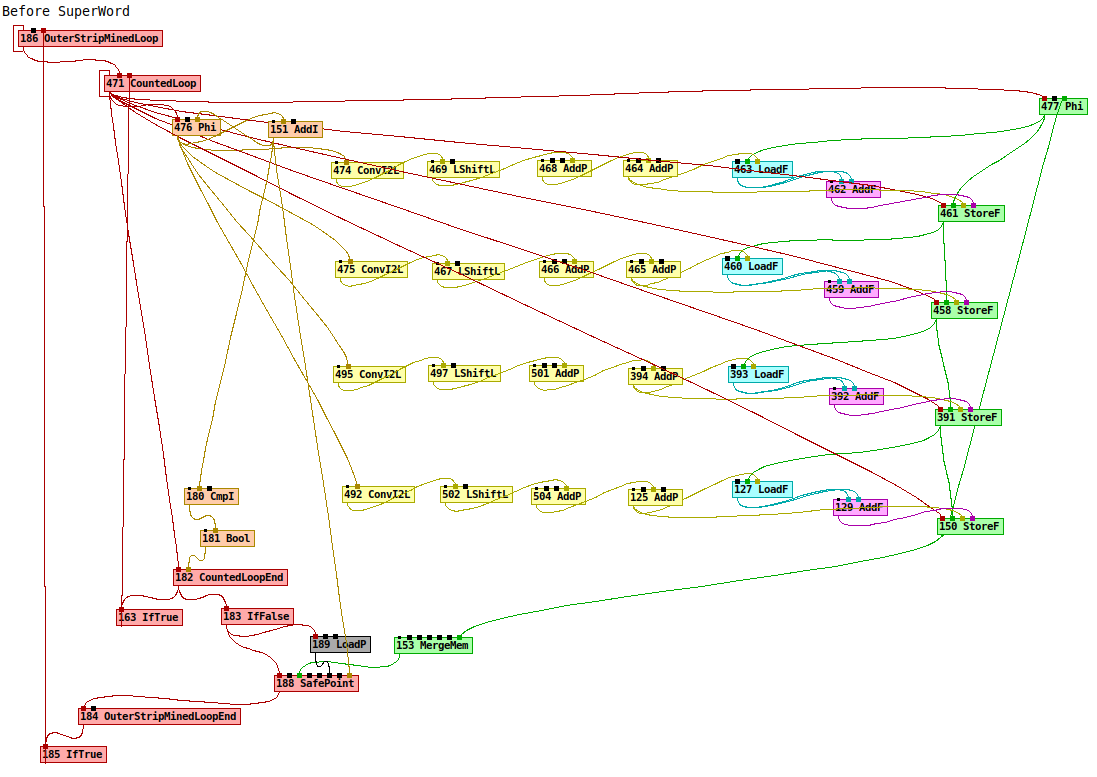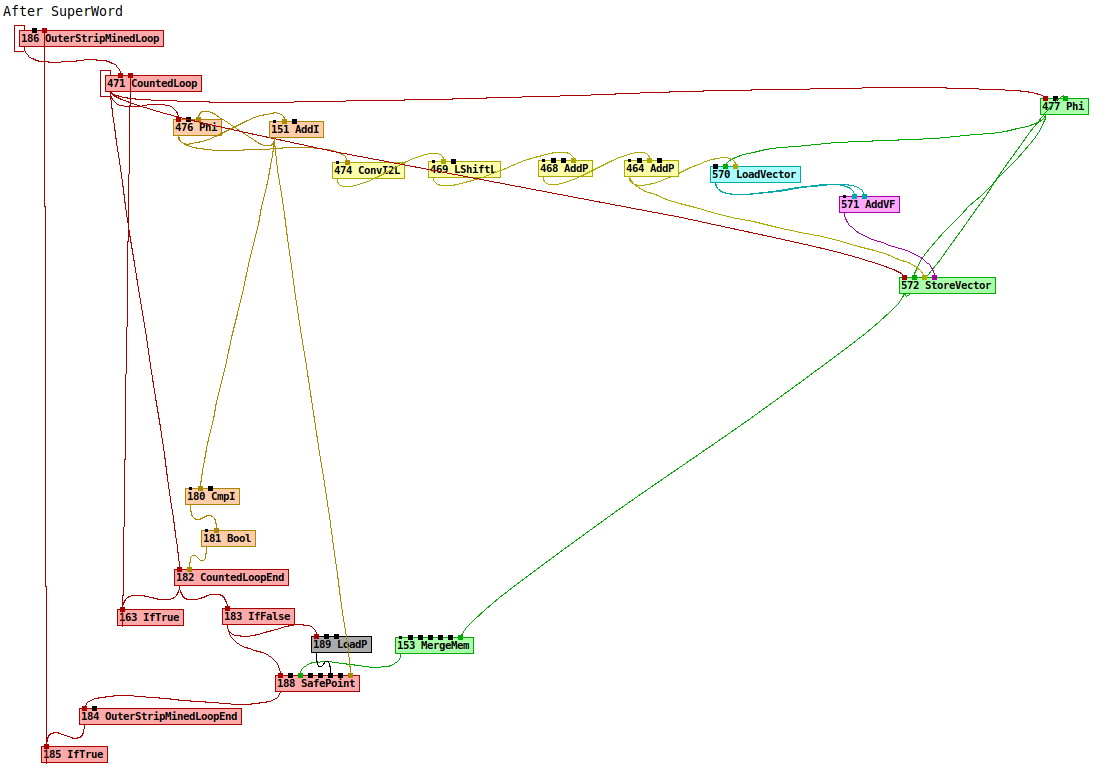One can see that the scalar operations were replaced with their vector equivalent:

``````LoadF -> LoadVector
AddF -> AddVF // Note: "2 * x" was replaced by "x + x"
StoreF -> StoreVector
``````

Basic Ideas for the Algorithm

We will look at the basic ideas of the algorithm on the same example loop, which we unroll twice (`4x` unrolled).

``````for (int j = 0; j < N; j++) { data[i] = 2 * data[i]; }
``````

I simplified the graph a little, but in essence the C2 graph looks like this: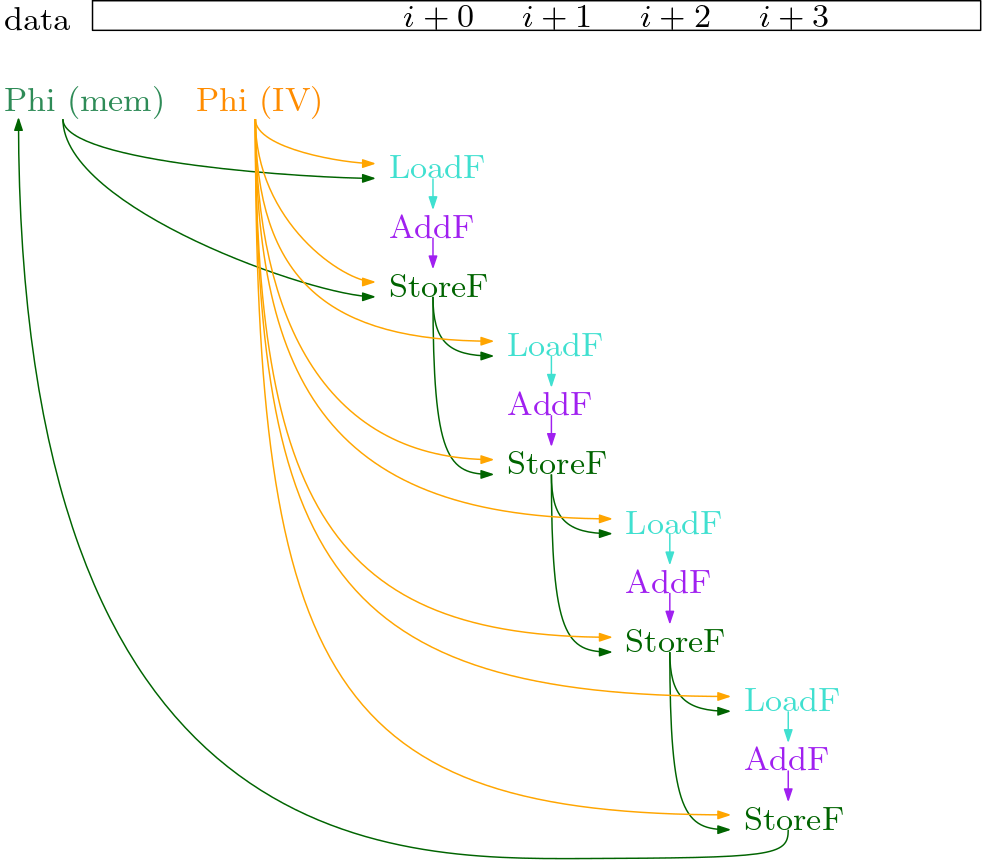We see the two `Phi` nodes: one holds the `i` (IV: induction variable), the other holds the memory state. I aligned all load, add and store operations with the respective offset in the `data` array. We can see that all the load and store operations here are on the same memory slice, of the float array `data`.

So far, we cannot see the parallelism in the graph. The `LoadF` of iteration `i+2` depends on the `StoreI` of iteration `i+1`. But we can prove that they do not access the same position in memory. Hence, we perform a dependency analysis, that gives us an improved dependency graph. In it, we ignore dependencies between loads and stores that do not access the same position in memory. In our example, we can remove all dependencies between the loop iterations.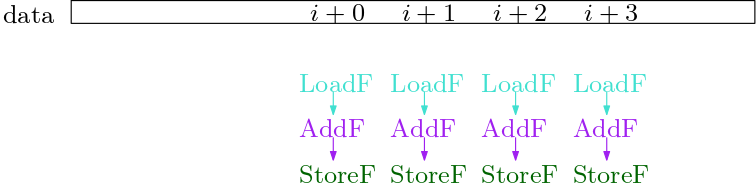Now, we see the parallelism in the dependency graph, that was apparent to the human eye when looking at the original Java code.

At this point, a few definitions and a more precise problem statement are due:

`DAG`: a `DAG` is a directed acyclic graph.

`Given`: the `DAG` with ops of a basic block (loop body, no control flow).

`Goal`: patch the `DAG` such that the scalar ops are packed into SIMD instructions. The new `DAG` must preserve the behavior of the old `DAG`.

`isomorphic`: to pack scalar ops into a single SIMD instruction, they must be similar (to simplify: same `Opcode` and `velt_type`).

`independent`: two ops are `independent` if there is no path from one to the other. We can only pack independent ops into a SIMD vector, since they are executed concurrently, so one cannot use the other’s output in any way.

`adjacent memory operations`: two memory operations that have a provable offset of exactly `sizeof(type)`. Two loads or two stores that are adjacent can thus potentially be packed into a single vector load or store (we can avoid using gather and scatter operations that make everything much more complicated).

`pack`: an `n-tuple` `[s1, ..., sn]`, where `s1, ..., sn` are `independent` and `isomorphic`.

`pair`: is a `pack` of size two.

`PackSet`: a set of `packs`.

Note: `isomorphism` and `independence` are necessary conditions for SLP vectorization. They are useful to make “local” decisions about individual nodes in their graph-neighbourhood. However, they are not sufficient to ensure that there are no cycles. We will discuss this in more details later.

At this point, we pack pairs of memory operations that are `adjacent`, `isomorphic` and `independent`. This is the initial `PackSet`.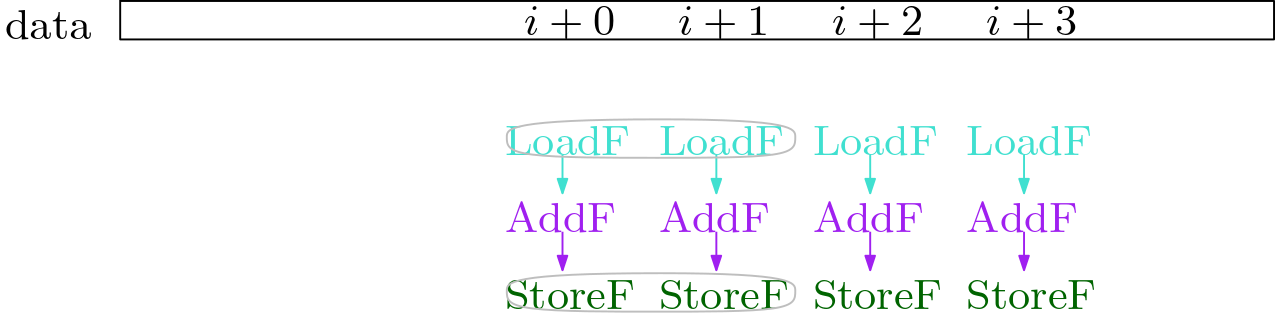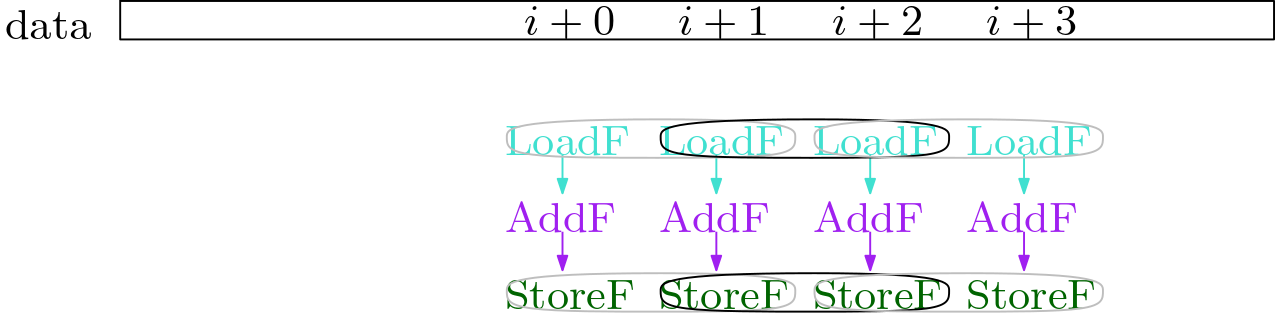Now we extend the `PackSet` from the memory operations to the non-memory operations. We do this by starting at a pair that we already have, and checking if the pair has an input pair, or an output pair that matches (ie. is `isomorphic` and `independent`).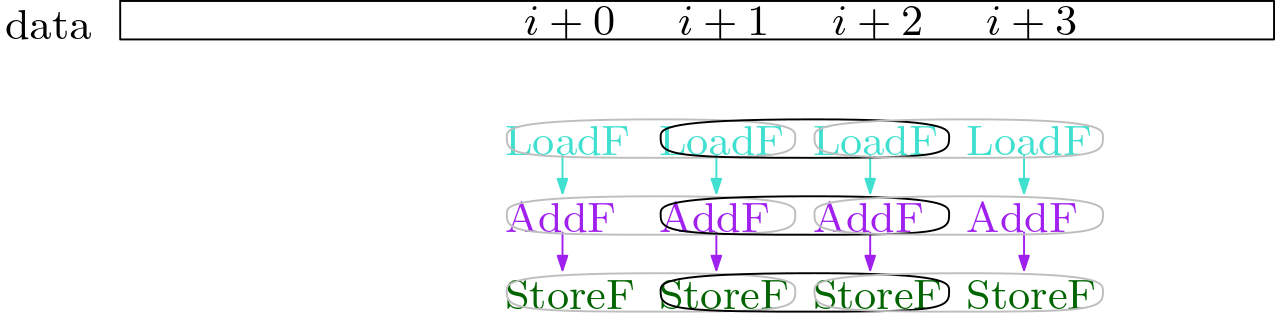Once we have found all pairs, we can combine the `pairs` into larger `packs`, by stitching them together: `[A, .., B] + [B, .., C] -> [A,.. B, .. C]`.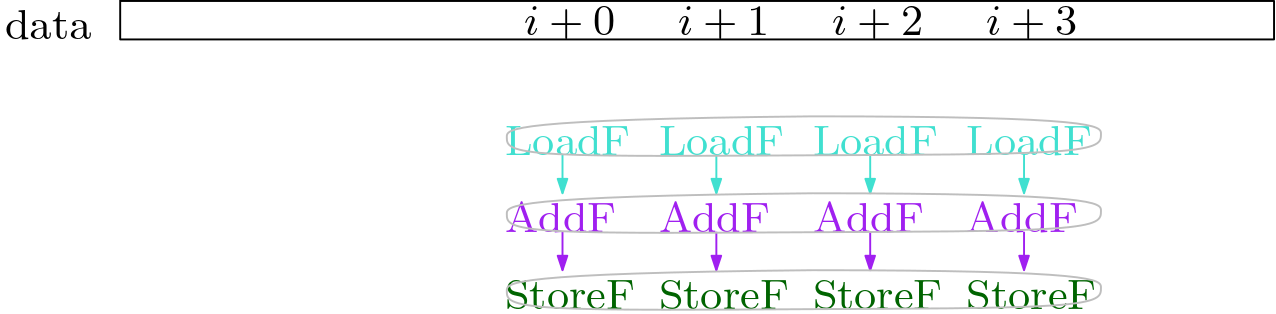At this point, we need to do some sanity checks, and determine if vectorizing is indeed profitable. Some `packs` or the `PackSet` will be filtered out. Finally, we can schedule the `PackSet`, and replace the C2 IR nodes that are in the `PackSet` with vector nodes.

Let’s look at two other examples. In the first, we store “backward” (`i-1`), in the second we store “forward” (`i+1`). In the first, the loop iterations are `independent`, while in the second, we see that the `StoreF` from the previous iteration stores to the position that the next iteration’s `LoadF` loads from. Such a depedency must be respected. Now, we see that the loads are `not independent`.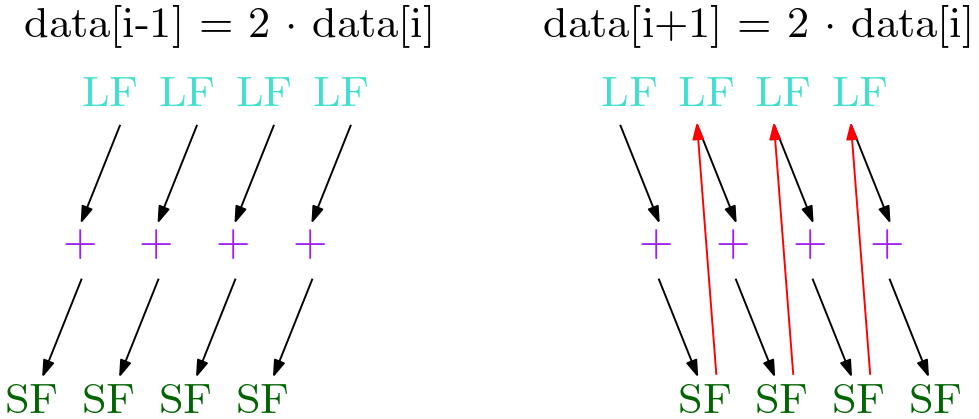These are the basic ideas used in the algorith and implementation. It may seem simple now, but the complexity lies in the details.

We will now look at each step of the algorithm in more detail.

Algorithm Step 0: Loop Unrolling

As already explained earlier, the algorithm works on basic blocks. This can be the body of a loop (without any control flow). But it can be any basic block, even outside of loops.

The implementation in the HotSpot JVM currently only applies the SuperWord algorithm for loops. Since it is a JIT (just in time) compiler, one has to focus the time spent on optimizations to the places where it is most promising. That is most often loops.

To ensure that the full size of the SIMD vectors can be filled, one needs to unroll loops with at least a multiple of `vector_width / sizeof(type)`. In the JVM code, this is further refined for each `type`. One will have to unroll less for an 8-byte `long`, than for a 2-byte `short`. Unrolling less means the algorithm has to process fewer noodes, and process them faster.

Still: the SLP algorithm also allows hand-unrolled loops (see example below).

``````for (int i = 0; i < RANGE; i+=2) {    // stride 2
dataF[i+0] = dataI[i+0] + 0.33f;  // i+0
dataF[i+1] = dataI[i+1] + 0.33f;  // i+1
}
``````

Algorithm Step 1: Alignment Analysis

Sadly, the paper handles this fairly quickly and without detail. It refers to another publication of the same authors, which I could not find. I had to look at the JVM implementation to understand it better. However, currently there are still bugs that are being fixed, where the alignment analysis is wrong, and other cases where it is too restrictive.

First, we need to extract the dependency graph from the C2 sea of nodes. Here we make use of the `memory slices` that were discovered by `Escape Analysis` (an algorithm that determines which memory accesses are related, and which are provably unrelated). We can ignore all inter-slice memory dependencies. Inside a memory slice, we need to ensure that `RAW` (read-after-write), `WAR` (write-after-read) and `WAW` (write-after-write) dependencies from the C2 graph are respected. But we can ignore `RAR` (read-after-read), since that is not a true dependency (swapping them has no effect). However, we can ignore `RAW`, `WAR` and `WAW` dependencies if they are provably accessing non-overlapping memory regions. The dependency graph now only consists of such `memory` edges, and all `data` edges from the C2 graph (dependencies `data -> data`, `data -> memop`, `memop -> data`).

In the JVM code, we represent memory addresses in the loop as follows:

``````address = base + stride*iv + const [+ invar]
``````

The `base` is associated with the base of an array reference. The `iv` references the induction variable `Phi`. `stride` is the distance in bytes between the loop iterations. `const` is a constant offset we have to the `base`. Optionally, there may be an `invar`, which is a value that is unknown, but invariant over all loop iterations. Given this, we can try to prove that memory accesses are non-overlapping, and we can potentially find the offset in bytes between two memory accesses, which helps us determine adjacent memory operations.

A second task is to ensure strict alignment on machines that require it (when the `AlignVector` HotSpot JVM flag is enabled). Many CPU’s require vector memory accesses to have a certain `X`-byte alignment in memory (eg. 4-byte or 8-byte). If a vector memory access is performed that is not `X`-byte aligned, this may lead to worse performance. Some CPU’s will also throw a `SIGBUS` error. And others simply ignore the lower bits, which leads to an access at a different location than intended (and hence to wrong results).

The JVM code picks one pack as the reference (`best`). All other pack have to be at an offset that aligns with `best`. We can then adjust the iteration count of the Pre-loop such that `best` is `X`-byte aligned to the memory. Since all other packsets are `X`-byte aligned relative to `best`, they then also `X`-byte aligned to the memory.

Note: currently the JVM code picks `X` to be the `vector_width` of the largest packset. This is suboptimal and should be improved.

Algorithm Step 2: Identifying Adjacent Memory References (create pair PackSet)

How should we pack the individual nodes into `packs`? The `adjacent` memory accesses are an obvious starting point, as we would like them to be in the same vector operation, in the correct order. We take an inductive approach, and seed the `PackSet` with `pairs of adjacent independent isomorphic` memory operations.

Assumption: “In practice, nearly every memory reference is directly adjacent to at most two other references.” One left, one right of it.

Further: duplicates should be removed by redundant load/store elimination (I guess that would be `LoadNode::Identity` and `StoreNode::Identity`).

Note: in the HotSpot JVM implementation, the `alignment analysis` is performed only at this stage, it is mixed into the same loop.

Algorithm Step 3: Extend PackSet (to non memory nodes)

Starting at the memory `pairs`, we extend the `PackSet` iteratively with non-memory `pairs`, until no new ones can be added.

• `follow_use_defs`: find `inputs`.
• `follow_def_uses`: find `outputs`.

The new `pairs` must be: `isomorphic` and `independent` (packable into SIMD vector instruction).

Side note: There is also a cost model that decides if packing it is profitable, and tries to extend in the most profitable way. It is also supposed to weight the gains made by executing operations in parallel, versus the potential costs of packing/unpacking if the inputs/outputs are not vectorizable. I have not investigated this much. I also fear that it is not very effective, because in the `filtering` stage we remove `packs` where the inputs would have to be packed, or the outputs unpacked.

Side note 2: We should only extend to non-memory nodes. If we also extend to memory nodes, then we may re-introduce loads/stores that we rejected, for example because of mis-alignment.

Algorithm Step 4: Combine PackSet (stitch the pairs together)

We now have all the `pairs`, that follow the `use-def` chains. We now iteratively stitch the `packs` together.

``````[s1, .., sj] + [sj, .., sn] -> [s1, .., sj, .., sn]
``````

Every node is now in maximally one `pack`. Any `pack` with a size other than a power of 2 is removed.

We split the `pack` into multiple if it is larger than the hardware would allow.

Detail: so far we have only shown that the `pairs` were `independent`. How do we know that the `packs` are now `independent`? `pair independence` still leaves room for dependence at distance `>=2`. For example `data[i+2] = 2 * data[i]` has a cyclic dependency at distance 2.

The paper states that `independence` is ensured during alignment analysis. It assumes that no `pair` is added that crosses an “alignment boundary”. More details are not provided.

In the JVM code, we currently do this as follows: If `AlignVector` is enabled, then we aready know that all vectors are aligned to the largest vector width. For the rest, we assume no alignment requirement by the hardware. `SuperWord::find_align_to_ref` finds the largest group of stores (or loads) with references “similar” to it. It then picks the reference with the smallest offset (the groups `mem_ref`). We do this by analyzing the address represented as `address = base + stride*iv + const [+ invar]`. We do this iteratively for all such groups. If two groups are in the same memory slice, we check if the two `mem_refs` are vector width aligned (same offset modulo vector width). This ensures that no `pair` inside a memory slice will cross this “alignment boundary”. This on its own would not really guarantee independence. However, in the JVM we do not implement packing/unpacking of vector-nodes, and also no vector-permutations. This means that every “vector-lane” stays independent.

However, if we use `-XX:CompileCommand=option,package.Class::method,Vectorize`, the flag `_do_vector_loop` is turned on. The `IntStream forEach()` method has this enabled implicitly (`vmIntrinsics::_forEachRemaining`). The `mem_ref` alignment check is disabled. Hence, we can create `pairs` that cross the “alignment boundary”. In that case, we cannot know if the `packs` are independent after the `combination`. We need an additional `independence` filtering on the `pack` level.

Algorithm Step 5: Filter Packset (implemented, profitable, cyclic dependencies)

This is an additional step that is not described in the paper, but implemented in the JVM. We check that all `packs` are:

• `implemented`: can we generate the required SIMD instruction? This is hardware dependent. The checks also query `Matcher::match_rule_supported_superword`. Consult `Matcher::match_rule_supported_vector` in `x86.ad` to see what vector instructions are implemented for which `SSE` and `AVX` CPU features.
• `profitable`: since the cost model was already applied during extension, we now only check if the `packs` can be connected to all inuts and outputs. There are a few open tasks stated in the comments.
• `cyclic dependencies`: `independence` on the `pack` level does not guarantee that there are no cyclic dependencies between the `packs`.

I quote from the paper:

``````3.7 Scheduling
Dependence analysis before packing ensures that statements within a group can be executed
safely in parallel. However, it may be the case that executing two groups produces a dependence
violation. An example of this is shown in Figure 6. Here, dependence edges are drawn between
groups if a statement in one group is dependent on a statement in the other. As long as there
are no cycles in this dependence graph, all groups can be scheduled such that no violations
occur. However, a cycle indicates that the set of chosen groups is invalid and at least one group
will need to be eliminated. Although experimental data has shown this case to be extremely rare,
care must be taken to ensure correctness.
``````

The idea is this: before `schedule`, we must ensure that `packs` are `independent`. But: `independence` on the `pack` level is not sufficient, we also need to ensure that the `packs` (groups) are `acyclic` before we `schedule`.

In the paper, they bring this example: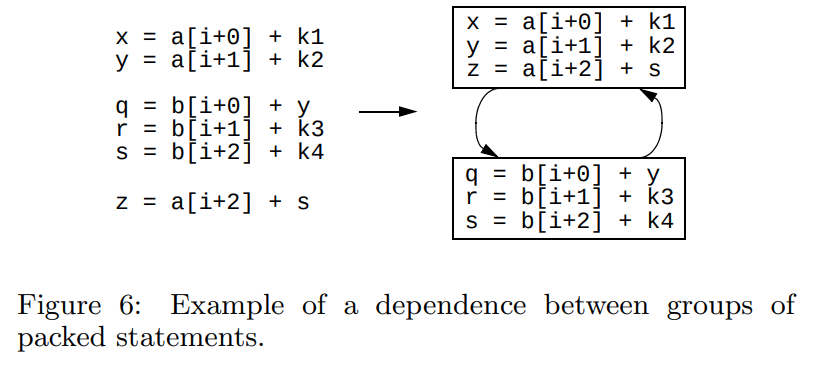This example does currently not get vectorized because it has “vector-lane” permutations, and the packs do not match up.

However, during this investigation, I found the following example. It currently gets vectorized incorrectly, and I filed a bug for it:

``````    static void test(int[] dataI1, int[] dataI2, float[] dataF1, float[] dataF2) {
for (int i = 0; i < RANGE/2; i+=2) {
dataF1[i+0] = dataI1[i+0] + 0.33f;            // 1
dataI2[i+1] = (int)(11.0f * dataF2[i+1]);     // 2

dataI2[i+0] = (int)(11.0f * dataF2[i+0]);     // 3
dataF1[i+1] = dataI1[i+1] + 0.33f;            // 4
}
}
``````

Note: `dataI1 == dataI2` and `dataF1 == dataF2`. I only had to use two references so that C2 does not know this, and does not optimize away load after store.

Lines 1 and 4 are `isomorphic` and `independent`. The same holds for line 2 and 3. We creates the packs `[1,4]` and `[2,3]`, and vectorize. However, we have the following dependencies: `1->3` and `2->4`. This creates a `cyclic dependency` between the two `packs`.

Conclusion: `independence` on the `pack` level is a necessary condition, but not sufficient. We must explicitly check that the `pack`-graph does not introduce any cycles, so that we can guarantee that the output `DAG` is indeed acyclic, with the same semantics (same effect/results).

Algorithm Step 6: Schedule (patch the graph)

In the meantime, I have rewritten the scheduling algorithm, please read my dedicated post here.

Implementation Overview

This is a quick overview of the HotSpot JVM SuperWord implementation, with a few comments:

``````// perform analysis to see how much a loop should be unrolled
// based on the types used and how many elements would fit in a vector
IdealLoopTree::policy_unroll_slp_analysis

// Simplified from code:
bool SuperWord::SLP_extract() {
// find memory slices
// construct reverse postorder (rpo) list of block members
// should we even vectorize? check if there is a store or reduction
if (!construct_bb()) {return false;}

// build dependence graph for each memory slice:
// for every two memops in slice, check if they
dependence_graph();

// Propagate narrower integer type back when upper bits not needed.
// Example: char a, b, c; a = b + c;
// The AddI gets velt_type char.
compute_vector_element_type();

// initial Packset: find adjacent, isomorphic, independent pairs of memops
// perform alignment analysis (currently under some construction/bugfixing)

// extend PackSet from memops to non-memops pairs
extend_packlist();

// stitch pairs together: [a, b] + [b, c] -> [a, b, c]
// split them into multiple if larger than max vector size
combine_packs();

// implemented?         -> depends on hardware
// profitable?          -> are all use and def in loop vectorizable?
// cyclic dependencies? -> do the packs introduce cyclic dependencies?
filter_packs();

// check if graph with packs has cycles. if yes -> remove all packs
remove_cycles();

// hack the graph: replace the scalar ops with vector ops
schedule();
output();
}
``````

These are the bugs that I am working on at the time of writing this blog:

• JDK-8298935: fix independence bug in create_pack logic in SuperWord::find_adjacent_refs
• JDK-8304042: C2 SuperWord: schedule must remove packs with cyclic dependencies

These are more tasks we could/should consider:

• Code has lots of open tasks (eg. implement PackNode and ExtractNode)
• I added a few recently:
• JDK-8302662: [SuperWord] Vectorize loop when value from last iteration is used after loop (Jatin)
• JDK-8302673: [SuperWord] MaxReduction and MinReduction should vectorize for int (Jatin)
• JDK-8302652: [SuperWord] Reduction should happen after loop, when possible (Emanuel?)
• JDK-8303113: [SuperWord] investigate if enabling `_do_vector_loop` by default creates speedup (Emanuel?)
• JDK-8300865: C2: product reduction in ProdRed_Double is not vectorized (Jatin)
• JDK-8287087: C2: perform SLP reduction analysis on-demand (Roberto)
• JDK-8255622: Combine all vectorization tests in one directory (Vladimir K?)
• JDK-8260943: Revisit vectorization optimization added by 8076284 (Vladimir K? some buggy code is just hard-disabled)
• Investigation: where do we not even start SuperWord where it could work? Where do we fail to vectorize during SuperWord? Can we find and fix these cases?
• Should we CMove more, to absorb control flow?
• Strided access? Gather / Scatter
• Investigate when / if / how FMA is working - only with `Math.fma`?
• More `independence` through more fine-grained `memory slices`? Speculative: two arrays of same type are separate objects?

Appendix: List of integrated SuperWord RFE’s

I will add more as I find more of them. Well you can of course dig for them yourself in the history

• JDK 8289422: Fix and re-enable vector conditional move
• JDK 8283091: Support type conversion between different data sizes in SLP
• JDK 8231441: AArch64: Initial SVE backend support
• JDK-8245158: C2: Enable SLP for some manually unrolled loops (Missing tests!)
• JDK-8192846: Support cmov vectorization for float
• JDK-8153998: Masked vector post loops
• JDK-8151573: Multiversioning for range check elimination
• JDK-8149421: Vectorized Post Loops
• JDK-8139340: SuperWord enhancement to support vector conditional move (CMovVD ) on Intel AVX cpu
• JDK-8135028: support for vectorizing double precision sqrt
• JDK-8129920: Vectorized loop unrolling (unroll again after SuperWord)
• JDK-8080325: SuperWord loop unrolling analysis
• JDK-8078563: Restrict reduction optimization (when it is profitable)
• JDK-8076284: Improve vectorization of parallel streams (`forEachRemaining`)
• JDK-8074981: Integer/FP scalar reduction optimization

Appendix: Other Work

• All you need is superword-level parallelism: systematic control-flow vectorization with SLP (2022)
• paper, youtube. Handle control flow using masked vector instructions. Loop fusion / co-iteration: every element represents a loop. Basically: flatten control flow to single block, by using CMove/select/blend when control flow merges, and masked load/stores.
``````int x;
If (condition) { x = v1; } else { x = v2; }

// translates to

c = condition; (true)
v1 = …;  (c)
v2 = …;  (!c)
x = Phi(c, v1, v2); (true)

// translates to

x = CMove(condition, v1, v2);

// vectorized

c_vec = condition[i : i + 4]; // vector of conditions
v1_vec = v1[i : i + 4]; // compute both values for true / false branch
v2_vec = v2[i : i + 4];
x_vec = blend(c_vec, v1_vec, v2_vec); // select from true / false branch
``````
• goSLP - Globally Optimized Superword Level Parallelism Framework (2018)
• paper, youtube. Statement packing using integer linear programming. Not JIT compatible.
• Look-ahead SLP: auto-vectorization in the presence of commutative operations (2018)
• paper, youtube. Lookahead to reorder commutative operations, to improve `isomorphism` and vectorize more.